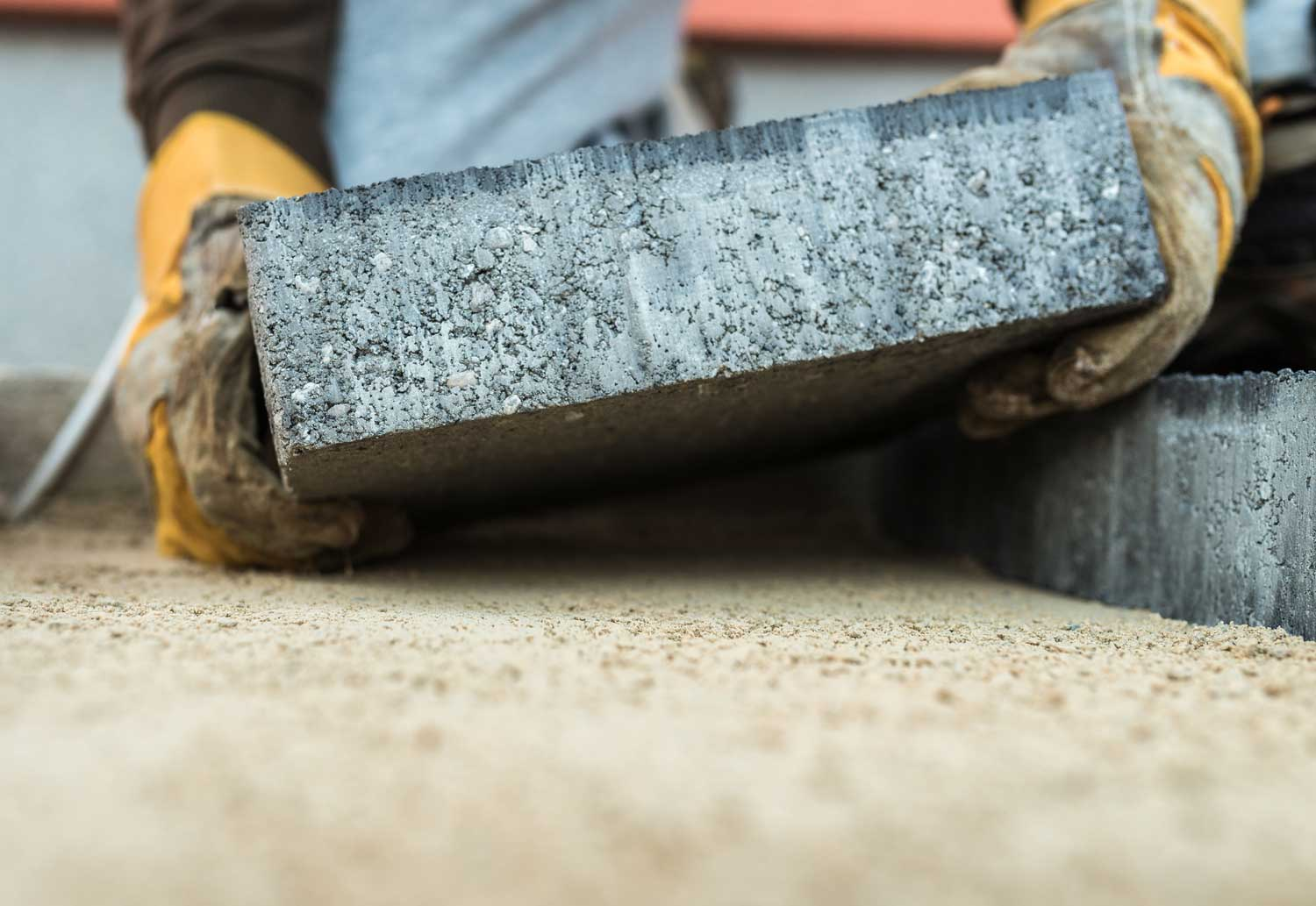# Paver Base Calculator

Calculate the amount of gravel and sand needed for the base for a paver patio, path, or driveway project. Enter the size of the project and desired depth of the base materials to get an estimate of gravel and sand required.

Project Size
Paver Base
Project Size
Find area using our square footage calculator
Paver Base

## Paver Base Material Estimate:

Gravel

cu yd

tons

Sand

cu yd

tons
Results account for 20% additional base gravel required for compaction.
Learn how we calculated this below

## How to Estimate Paver Base Sand and Gravel

A beautiful and long-lasting paver patio, driveway, or walkway starts with a solid base. A paver base consists of a layer of compacted crushed gravel topped with a layer of sand.

A solid base prevents the pavers from shifting, sinking, and upheaving. Make sure to start your paver project off right by having the correct amounts of base material on hand.### How to Find Cubic Yards of Base Material

To estimate the cubic yards of gravel needed for a paver base, follow these steps:

• Step One: Measure the length and width of the patio in inches
• Step Two: Multiply the length and width to find the area in square inches
• Step Three: Multiply the area by the gravel depth in inches to find the volume in cubic inches – traditional pavers are generally set on a 4″ – 6″ compacted gravel base
• Step Four: Divide cubic inches by 46,656 to find the amount of gravel in cubic yards

You can also use a gravel calculator to estimate this material.

To estimate the correct amount of sand, you need your area in square inches that you found by multiplying the length and width in inches. The layer of sand only needs to be 1 inch deep to prevent the pavers from moving, so the area in square inches is equal to the volume in cubic inches. Just divide that by 46,656 to find the number of cubic yards of sand required for the job.

### How to Find Cubic Meters of Base Material

Finding the cubic meters of gravel needed is very similar to the steps above, with slight changes:

• Step One: Measure the length and width of the patio in centimeters
• Step Two: Multiply the length and width to find the area in square centimeters
• Step Three: Multiply the area by the gravel depth in centimeters to find the volume in cubic cm
• Step Four: Divide the volume in cubic cm by 1,000,000 to find the amount of gravel in cubic meters

To estimate the correct amount of sand, you need your area in square centimeters that you found by multiplying the length and width in centimeters. Multiply the area in square centimeters by the desired sand depth in centimeters to find the volume in cubic centimeters. Then, divide that by 1,000,000 to find how much sand is needed in cubic meters.

## How Deep Should a Paver Base Be?

For a typical patio, a paver base should consist of a 4″ – 6″ layer of compacted gravel with a 1″ layer of sand. For heavier-duty applications such as commercial spaces or driveways, a 6″ to 8″ layer of gravel should be used.

Keep in mind that compacting your gravel will cause the initial amount to shrink. So if you need a 4″ base, you should estimate an additional 20% of material so that when compacted, it reaches the correct depth.

## Amount of Paver Base Material Needed for a Patio

Amount of paver sand and gravel base material needed for various patio or walkway sizes
Patio Size Gravel Needed
(4″ – 6″ base)
Sand Needed
(1″ base)
50 sq. ft. 0.75 – 1.12 cu. yds. 0.15 cu. yds.
100 sq. ft. 1.48 – 2.22 cu. yds. 0.31 cu. yds.
150 sq. ft. 2.22 – 3.34 cu. yds. 0.46 cu. yds.
200 sq. ft. 2.96 – 4.44 cu. yds. .62 cu. yds.
250 sq. ft. 3.71 – 5.56 cu. yds. 0.77 cu. yds.
300 sq. ft. 4.44 – 6.67 cu. yds. 0.93 cu. yds.
350 sq. ft. 5.18 – 7.78 cu. yds. 1.08 cu. yds.
400 sq. ft. 5.93 – 8.89 cu. yds. 1.23 cu. yds.
450 sq. ft. 6.67 – 10.01 cu. yds. 1.39 cu. yds.
500 sq. ft. 7.4 – 11.11 cu. yds. 1.54 cu. yds.
550 sq. ft. 8.15 – 12.23 cu. yds. 1.7 cu. yds.
600 sq. ft. 8.89 – 13.34 cu. yds. 1.85 cu. yds.
650 sq. ft. 9.62 – 14.45 cu. yds. 2.01 cu. yds.
700 sq. ft. 10.67 – 15.56 cu. yds. 2.16 cu. yds.
Next Step – Estimate how many pavers are needed using our paver calculator and estimate the amount of polymeric sand needed using our polymeric sand calculator.